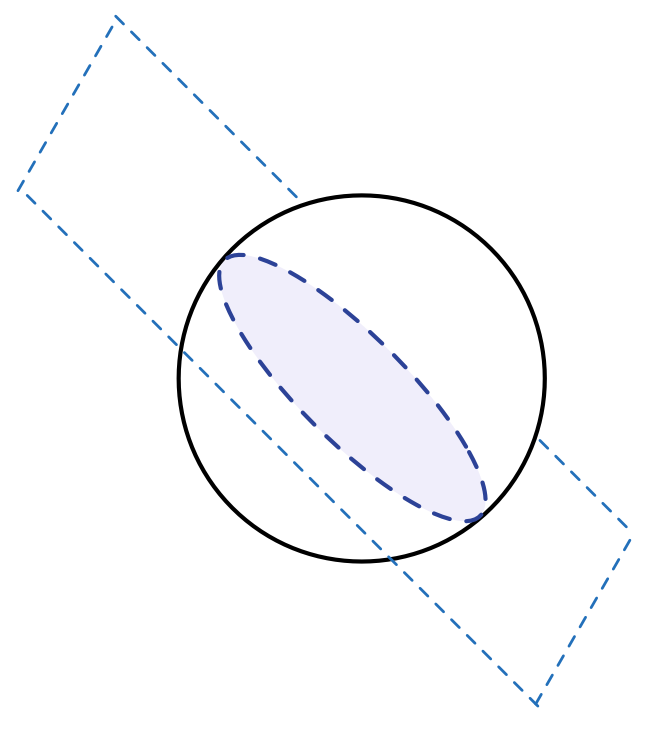# A Plane, A Sphere And A Hyperbola Walked Into A Bar

Algebra Level 4$x, y,$ and $z$ are real numbers such that $x+y+z= 0$ and $x^2 + y^2 + z^2 = 1$. What is the smallest possible value of $xyz$?

×

Problem Loading...

Note Loading...

Set Loading...